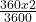## A car starts to move from rest and covers a distance of 360m in one minute. Calculate the acceleration of the car. ​

Question

A car starts to move from rest and covers a distance of 360m in one minute. Calculate the acceleration of the car.

in progress 0
5 months 2021-08-30T06:35:56+00:00 1 Answers 2 views 0

1. ## The acceleration of car is 0.2 ms⁻²

Explanation:

When the car moves , the distance covered is calculated by the relation

S = u t +a t²

In this question u = 0 , because car was at rest initially

Thus S =a t²

here S is displacement and a is the acceleration of car

Therefore  360 =a ( 60 )²

Because time taken is one minute or 60 seconds

Therefore a =or a = 0.2 m s⁻²